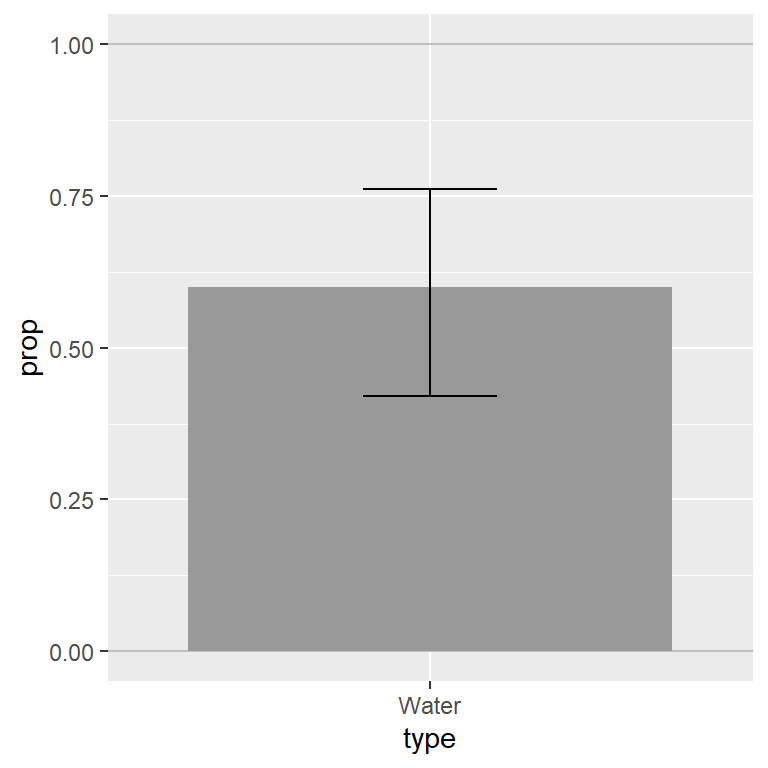# ADA1: Class 16, Parameter estimation (one-sample)

Advanced Data Analysis 1, Stat 427/527, Fall 2022, Prof. Erik Erhardt, UNM

Author

Published

August 13, 2022

# Rubric

Answer the questions in this document, compile to html, print to pdf, and submit to UNM Learn. Do not add this to your “ALL” .Rmd document.

# Sample the Globe example

How can we estimate the proportion of water on the globe using a beach ball?

1. (0 p) What is a good sampling strategy to pick points at random from a sphere?

In previous classes we brainstorm a strategy as we look at a beachball of the globe.

• Suggestions
1. Some suggest sampling latitude and longitudes, but those are not uniformly distributed on the earth and the poles would be sampled more densely than the equator.
2. Some suggest cutting the ball into pieces and measuring how much water is on each piece.
3. Finally, I suggest that we toss the ball around the room and when you catch it, look at your right pointer finger and determine if it’s on water or land; tossing randomizes the orientation of the ball, and catching samples a point on the ball.
1. (3 p) How can this strategy be used to estimate the proportion of the globe covered by water?

Assuming we use Strategy 3, … [answer here]

1. (0 p) Below are the data that we collected in class from a previous year. Compute the confidence interval for the true proportion of water on the ball.

Let $$n=$$ the total number of observations and let $$x=$$ the number of “successes” (number of water observations, land is a “failure”). These numbers are entered into the prop.test() and binom.test() functions below.

library(tidyverse)
── Attaching packages ─────────────────────────────────────── tidyverse 1.3.2 ──
✔ ggplot2 3.3.6     ✔ purrr   0.3.4
✔ tibble  3.1.8     ✔ dplyr   1.0.9
✔ tidyr   1.2.0     ✔ stringr 1.4.0
✔ readr   2.1.2     ✔ forcats 0.5.1
── Conflicts ────────────────────────────────────────── tidyverse_conflicts() ──
✖ dplyr::lag()    masks stats::lag()
## notes for prop.test() and binom.test()
# x = number of "successes"
# n = total sample size

x = 21
n = 21 + 14

dat_globe <-
tribble(
~type   , ~freq , ~prop
, "Water" ,     x ,      x  / n
, "Land"  , n - x , (n - x) / n
)
dat_globe
# A tibble: 2 × 3
type   freq  prop
<chr> <dbl> <dbl>
1 Water    21   0.6
2 Land     14   0.4
# prop.test() is an asymptotic (approximate) test for a binomial random variable
p_summary <- prop.test(x = x, n = n, conf.level = 0.95)
p_summary

1-sample proportions test with continuity correction

data:  x out of n, null probability 0.5
X-squared = 1.0286, df = 1, p-value = 0.3105
alternative hypothesis: true p is not equal to 0.5
95 percent confidence interval:
0.4220904 0.7564794
sample estimates:
p
0.6 
# binom.test() is an exact test for a binomial random variable
b_summary <- binom.test(x = x, n = n, conf.level = 0.95)
b_summary

Exact binomial test

data:  x and n
number of successes = 21, number of trials = 35, p-value = 0.3105
alternative hypothesis: true probability of success is not equal to 0.5
95 percent confidence interval:
0.4211177 0.7612919
sample estimates:
probability of success
0.6 
1. (4 p) Interpret the confidence interval for the proportion of water.

1. (3 p) Here’s a gimme! Label the plot: the title, $$x$$-axis, and $$y$$-axis.
Note

Note how to add error bars using geom_errorbar(). First determine the CI bounds from the binom.test() previously, then set those as limits.

# get names of objects in b_summary
names(b_summary)
 "statistic"   "parameter"   "p.value"     "conf.int"    "estimate"
 "null.value"  "alternative" "method"      "data.name"  
# here's the confidence interval bounds (the attribute tells us this is a 95% interval)
b_summary$conf.int  0.4211177 0.7612919 attr(,"conf.level")  0.95 b_summary$conf.int
 0.4211177
b_summary$conf.int  0.7612919 library(ggplot2) p <- ggplot(data = dat_globe %>% filter(type == "Water"), aes(x = type, y = prop)) p <- p + geom_hline(yintercept = c(0, 1), alpha = 1/4) p <- p + geom_bar(stat = "identity", fill = "gray60") p <- p + geom_errorbar(aes(min = b_summary$conf.int, max = b_summary\$conf.int), width=0.25)
print(p)